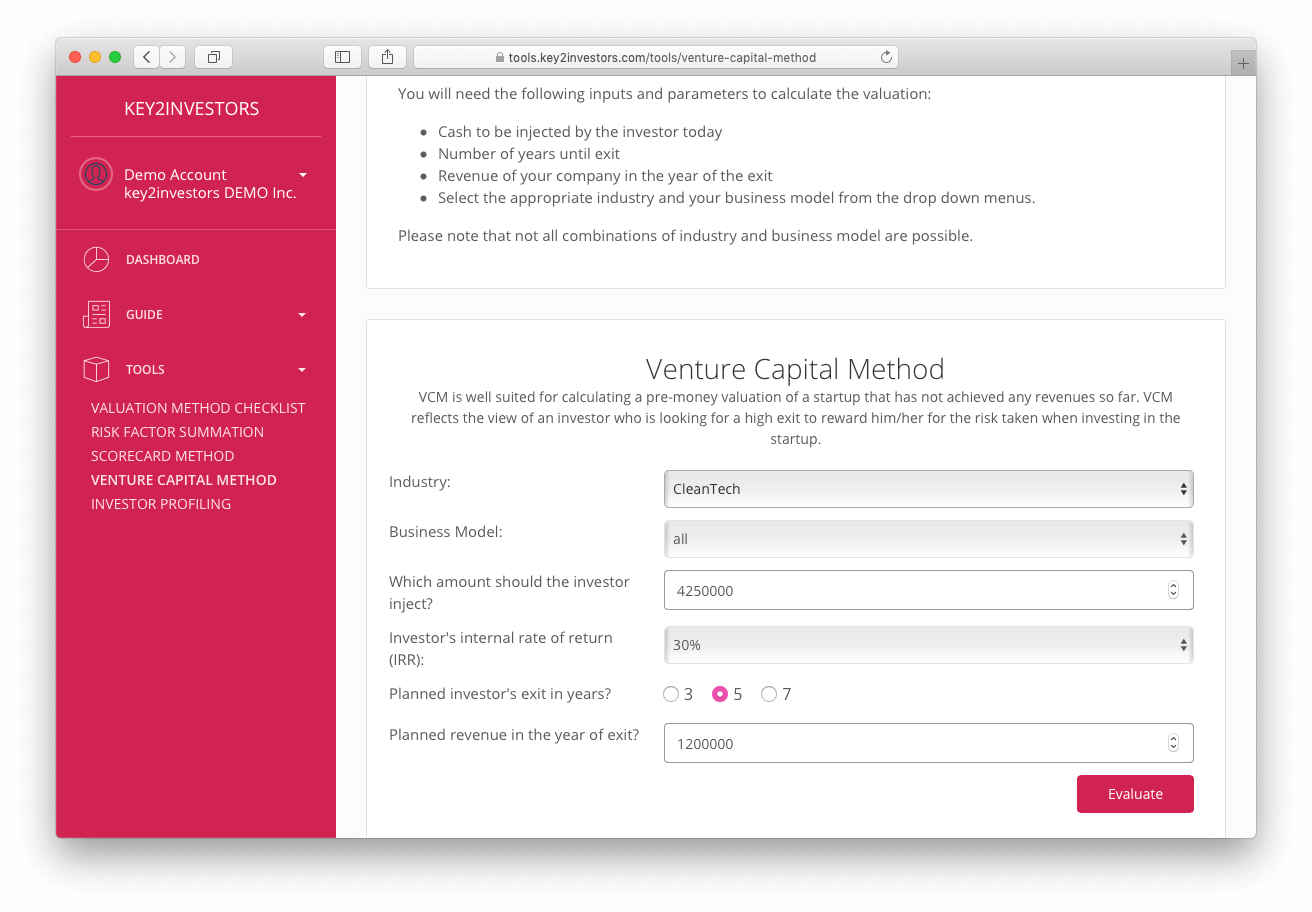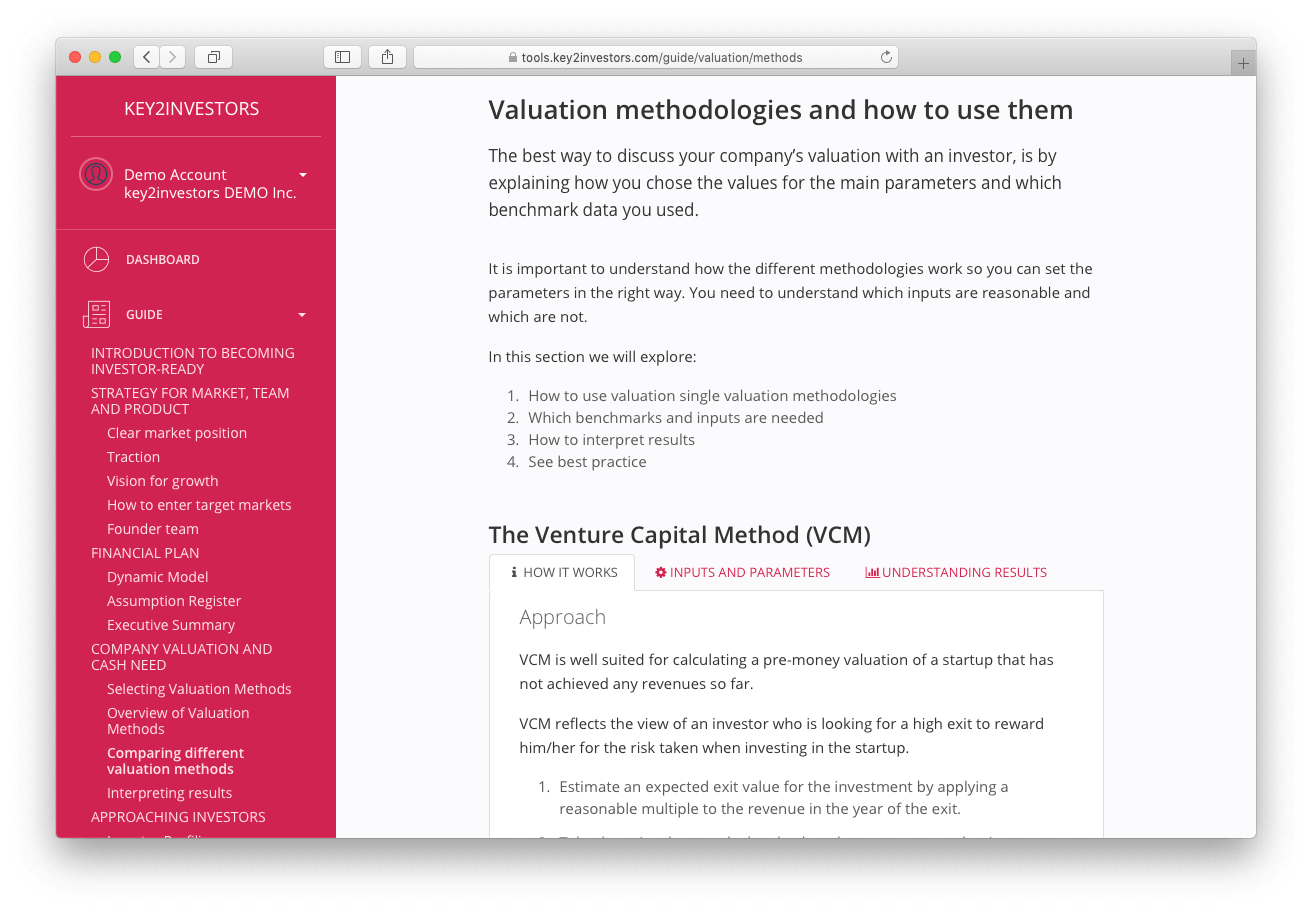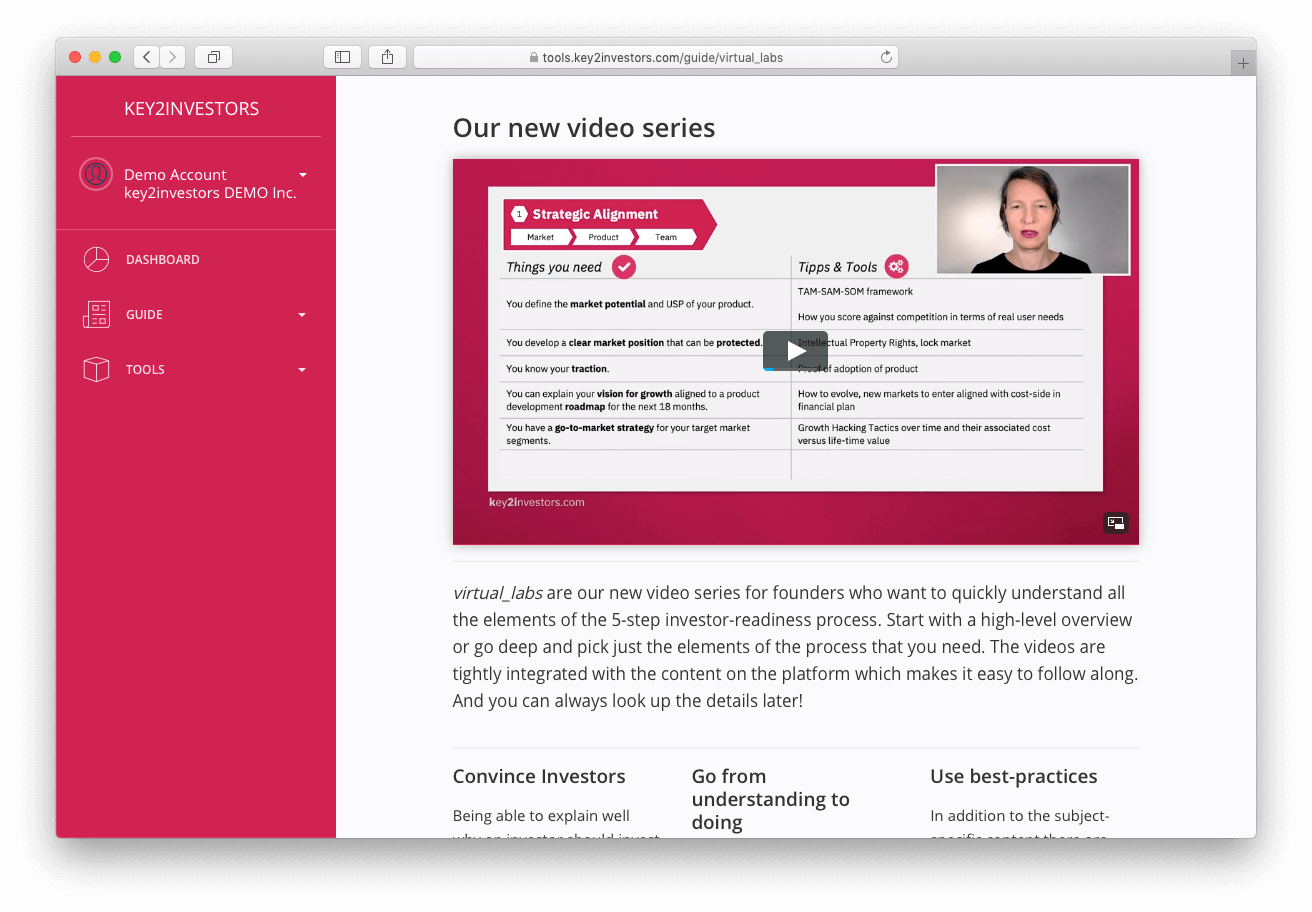# Startup Valuation Calculator

Find out which valuation calculator is best for your startup.
Get your startup’s valuation calculated with a trusted tool.
Based on startup valuation templates.

## Calculate my startup valuation range

When you sit down with an investor during your negotiation, you want to prepare. You need to have a robust understanding of the startup valuation process. You need to define the underlying valuation assumptions. This enables you to discuss at eye’s level with investors. At a certain moment during negotiations, you will discuss the startup valuation range. You will derive it by using different startup valuation calculators with different methods. The pre money valuation calculators for startups are:

• Risk Factor Summation Valuation Calculator
• Scorecard Valuation Calculator
• Venture Capital Valuation Calculator
• Discounted Cash-Flow Valuation Calculator
• First Chicago Valuation Calculator

On our platform we provide a set of tools based on startup valuation templates. The pre money valuation calculators allow you to calculate a valuation range.## Startup Valuation Calculators explained

You can learn more about the different startup valuation templates and calculators. We provide in the Guide section the chapters Company Valuation and Cash Need. There we cover these key points:

• Understand how different valuation calculators wor
• Being able to select the right pre money valuation calculator
• Researching benchmark data for startup valuations
• Understanding results and doing sanity checks
• Calculating a startup valuation range

## Get training on pre money valuation calculation

Learn about the different valuation techniques for fundraisers with our masterclass videos. Use the valuation calculators to get a plausible valuation range for your startup. Learn how to derive benchmark data and how to interpret the results.## Frequently Asked Questions on startup valuation calculators

#### Isn’t the valuation of my startup fixed when I am early stage?

When you are pre-seed and pre-product, your valuation is somehow fixed. Depending on your team and the market potential, it can vary a little. Once you are in seed, you got a working prototype, the situation changes. Valuations differ depending on some factors. These can be

• the team
• the market expectations

Using a startup valuation calculator helps you in understanding the key assumptions. They are important to derive a higher valuation for your startup. You need good arguments for your valuation.

#### How does a pre money valuation calculator differ from a post money valuation calculator?

Pre money valuation is the valuation of your startup before an investor puts money in. If you add the funds raised from an investor to the pre money valuation, you get the post money valuation. It is better to negotiate pre money valuations with investors. This is the reason why you are looking for pre money valuation calculators. Yet, it is easy to get from a post money valuation to the pre money valuation. Reduce the post money valuation by the funds raised from your future investor and there you go.

#### What is a startup valuation range?

Valuations of startups are a quite subjective estimate. Opinions can differ. Still, the final valuation used for an investor to step in, should be between x and y. This is the valuation range. It is limits the lower and the upper end of an acceptable valuation.

#### Why do I need a startup valuation calculator?

If we run calculations for the first time, it gives us comfort to use a trusted tool that an expert tested before. There should not be mis-calculations for this sensitive topic of startup valuations. Moreover, a startup valuation calculator shows the results. It puts them into perspective. This gives security that valuations are not far off. The valuation calculator for startups helps to structure arguments. So you can defend your level of valuation. It helps you to compare to other startups’ valuations. Good startup valuation calculators provide data. You need it to benchmark and to have a good starting point for your analysis.

### Our partners share our values and support startups